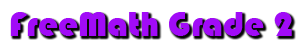Counting and Writing Numbers 1A Counting to 100 1B Counting to 200 1C Counting by 2s Using Pictures 1D Counting by 5s Using Pictures Addition Facts 1E Write a Number Sentence Using Pictures 1F Sums to 6 1G Sums to 10 1H Sums 11 to 18 Subtraction Facts 1I Use Pictures Write Subtraction Sentence 1J Single Digits Addition and Subtraction 2A Adding Three Numbers 2B Order Property and Zero Property 2C Addition Patterns, Math Rule 2D Problem Solving, How Many in All 2E Problem Solving, Draw a Picture 3A Subtraction, 2-Digit Minus 1-Digit, Horizontal 3B Subtraction, 2-Digit Minus 1-Digit, Vertical 3C Problem Solving, Subtraction, Draw a Picture 3D Problem Solving, Subtraction, How Many More 3E Missing Addends, Use a Related Subtraction Fact 4A Addition, 2-Digit Plus 1-Digit, Horizontal 4B Addition, 2-Digit Plus 1-Digit, Vertical 4C Fact Families 4D Problem Solving, Draw a Picture Place Value 5A Write How Many Tens and Ones 5B Add by Combining Blocks 5C Circle the Value of the Underlined Digit Writing  Numbers and Number Words 5D Writing Number Words: 1 to 20 5E Number Words: 20 to 100 Compare and Order Numbers 6A Comparing Numbers to 30 6B Comparing Numbers 30 to 100 6C Missing Numbers on a Number Line to 50 6D Missing Numbers on a Number Line to 100 6E Order Numbers, Before, After, Between 6F Skip Count by 5s 6G Skip Count by 10s 6H Odd and Even Numbers Money 7A Pennies, Write the Total As You Count 7B Pennies and Nickels 7C Pennies, Nickels and Dimes 7D Pennies, Nickels, Dimes and Quarters 7E Coin Value to Half Dollar 7F Adding Coins to Match a Price 7G Different Combinations for the Same Amount 8A Counting Dollar Bills and Coins 8B Enough Money to Buy and Item 8C Compare Coin Amounts 8D Money Equivalents 8E Use the Fewest Coins 8F Making Change 8G Add Money 8H Subtract Money 8I Problem Solving, Subtraction Time 9A Read a Clock to the Hour 9B Time to the Half Hour 9C Time to the Quarter  Hour 9D Read a Clock to 5 Minutes 9E Draw Hands on a Clock 9F Write Time More Than One Way 9G Problem Solving, Time, Using a Model 9H Estimate Time, One Minute 9I Estimate Time 10A Days of the Week 10B Sequencing Months 10C Using a Calendar 10D Days, Weeks, Months, Years Graphs 11A Graphs, Pictograph 11B Read a Tally Table 11C Make a Bar Graph 11D Read a Line Graph 12A Place Value, Tens and Ones 12B Place Value, Tens and Ones 12C Place Value, Tens and Ones Addition and Subtraction With Regrouping 13A Addition 2-Digit, Regrouping Ones 13B Problem Solving, Addition 14A Addition, Rewrite a Number Sentence 14B Adding 3 Numbers 14C Estimate Sums 14D Add and Estimate 15A Subtraction, Count Back 10s to Subtract 15B Subtraction, Differences From Ten 15C Subtraction, 2-Digit, No Regrouping 15D Subtraction, 2-Digit, With Regrouping 15E Problem Solving, Subtraction 15F Problem Solving, Subtraction, How Many More 16A Subtraction, Add to Check 16B Subtraction, Estimate to Check Measurement 17A Length in Inches 17B Print and Use an Inch Ruler 17C Length in Centimeters 17D Print and Use a Centimeter Ruler 17E Nonstandard Units 17F Estimate and Measure to the Nearest Inch 17G Inch, Foot, Yard 17H Problem Solving, Length 18A Kilograms 18B Pounds 18C Ounces 18D Capacity, Cups, Pints, Quarts 18E Capacity, Quart, Gallon 18F Capacity, Milliliter, Liter Geometry 19A 3-Dimensional Figures 19B Name 3-Dimensional Figures 19C 2-Dimensional Shapes 19D 3-Dimensional Shapes 19E Hexagon, Trapezoid 19F Parallelogram 19G Name the Shape 20A Faces, Edges, Vertices 20B Geometric Patterns 20C Area 20D Congruence 20E Symmetry 20F Slide, Flip, Turn 20G Perimeter Place Value 21A Place Value 21B Place Value 21C Problem Solving Compare and Order Numbers 22A Order Numbers 22B Compare Numbers 22C Order Numbers, Least to Greatest 22D Order Numbers, Greatest to Least Number Patterns 22E Find the Pattern, Write the Missing Number 22F Count Forward and Backward 22G Write the Missing Numbers Addition; 3-Digit With Regrouping 23A 3-Digit Numbers, Adding Hundreds 23B 3-Digit Numbers, No Regrouping 23C 3-Digit, Regrouping Ones as Tens 23D 3-Digit, Regrouping Tens as Hundreds 23E Problem Solving Subtraction; 3-Digit With Regrouping 24A Subtracting Hundreds 24B 3-Digit, No Regrouping 24C 3-Digit, Regrouping Ones as Tens 24D 3-Digit, Regrouping Tens as Hundreds 24E Subtract Money, Estimate to Check 24F Problem Solving, Choose the Operation Fractions 25A Halves, Circle Shape That Shows Halves 25B One Half, Color One Half 25C Thirds, Circle Shape That Shows Thirds 25D One Third, Color One Third 25E Fourths, Circle Shape That Shows Fourths 25F One Fourth, Color One Fourth 25G Color One Part and Identify 25H Connect Dots, Write Fraction for Shaded Part 25I Problem Solving, Fractions 25J Compare Fractions Probability 26A Probability 26B Likely, Unlikely 26C Predict 26D Combinations 27A Using Data, Range, Median and Mode